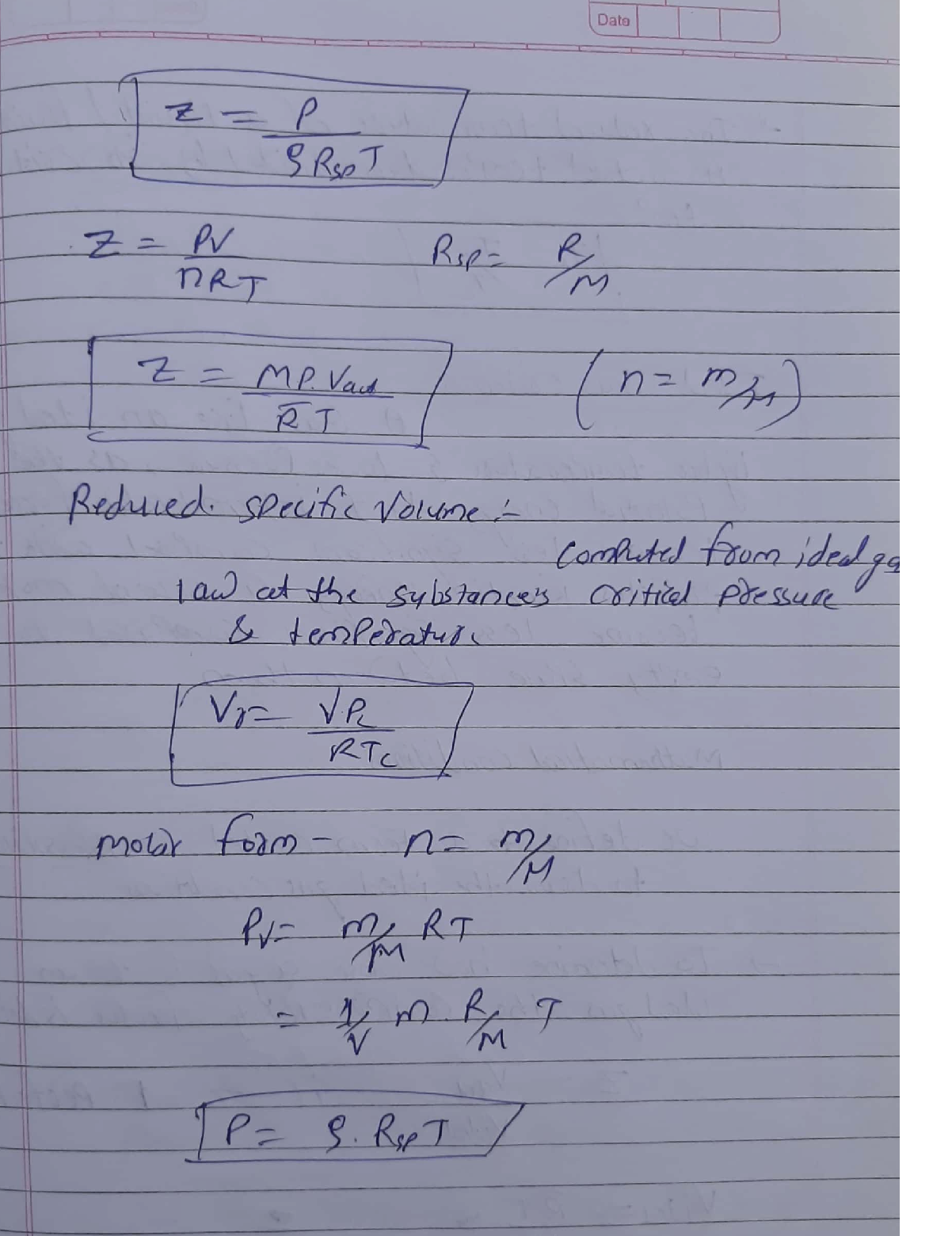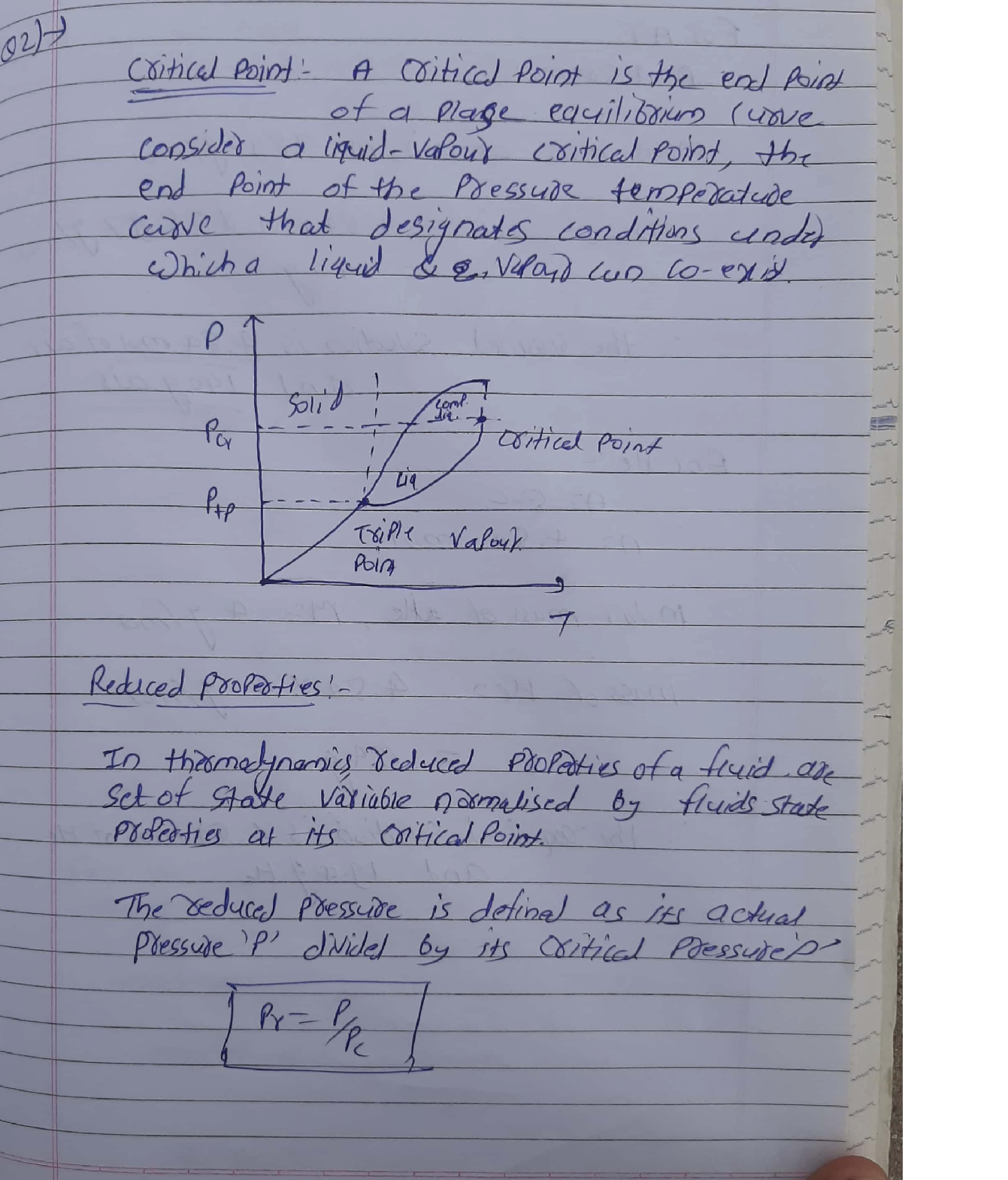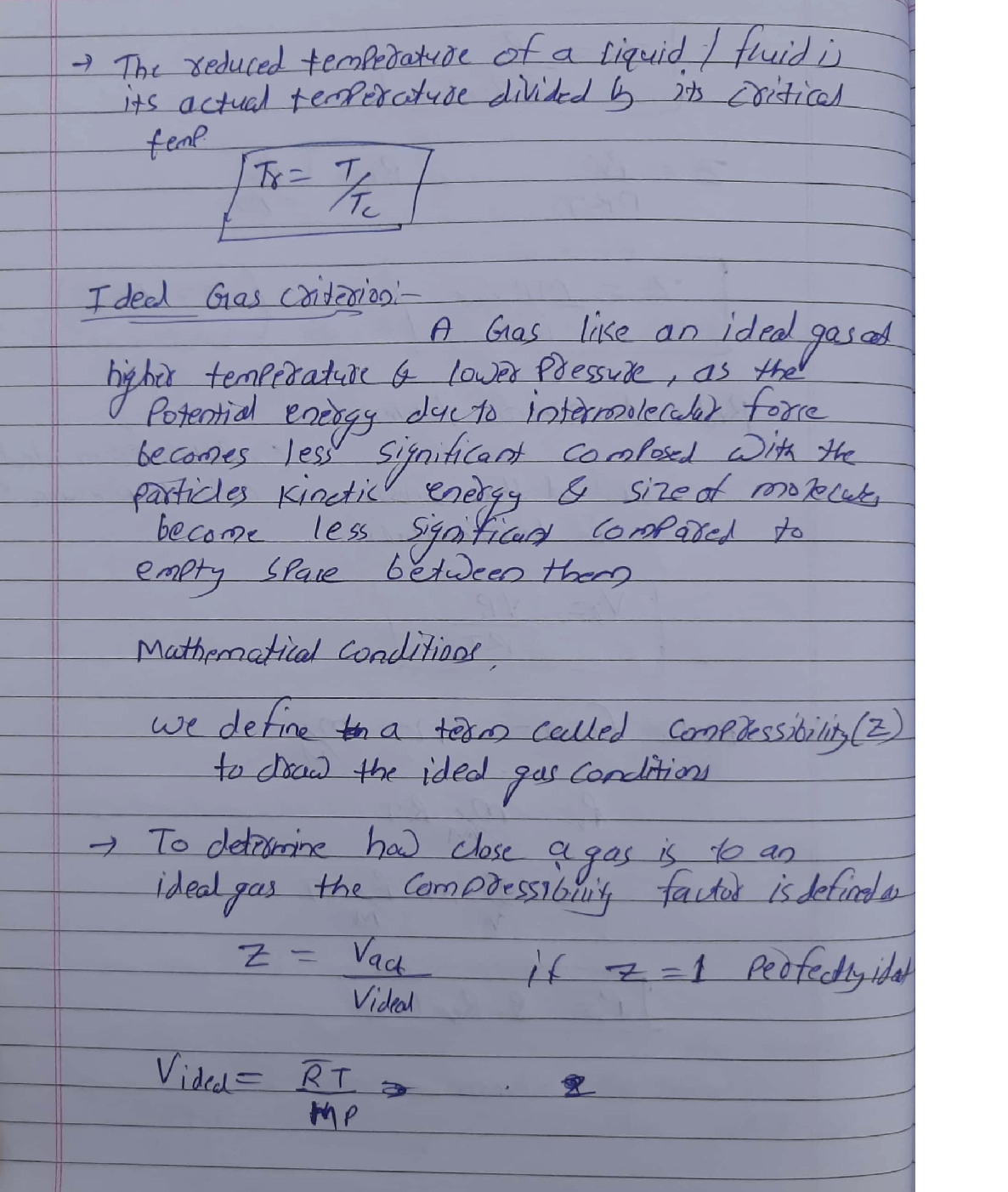Question

Heat Transfer

2. (20 pt) What does critical point mean? What are reduced pressure and temperature? What are the ideal gas criteria?Explain physically based on reduced pressure and temperature. Also give its mathematical conditions.Verified### Question 45577Heat Transfer

Problem 3: In a molten-salt nuclear reactor, radioactive liquid is flowing through a cylindrical pipe of diameter D. Fission in the liquid results in a uniform internal heat generation of ġ W/m3. The pipe wall also has a heater on it that can provide a uniform surface heating rate of q" W/m². The radioactive liquid has a specific heat capacity of Cp J/kg-K, a mass flow rate of ṁ kg/s, and an inlet temperature of Tmi.Conduction in the fluid in the x-direction can be assumed to be negligible, conduction through the pipe wall is negligible, and the flow in the pipe is steady and fully developed. Using a differential control volume in the pipe, perform an energy balance to obtain an ordinary differential equation that describes the variation of the bulk temperature (Tm) as a function of x-distance along the pipe. What is the boundary condition for this differential equation?

### Question 45576Heat Transfer

Problem 2: A cylindrical capacitor mounted to a circuit board in an AC-to-DC power converter is initially at a uniform temperature of 55°C. The capacitor has a diameter of 1 cm and a length of 1.5 cm, with a density of 5000 kg/m³, a specific heat capacity of 3 kJ/kg-K, and a thermal conductivity of 8 W/m-K. A fan blows air at a velocity of 5 m/s and 20°C past the capacitor. If the power converter is turned off (assume the fan continues to run), how long would it take for the capacitor temperature to drop below 30°C? Ans:9.8 min

### Question 45575Heat Transfer

Problem 1: You are designing a nuclear fuel element for a water-cooled nuclear reactor. The element is shaped like a flat plate, with length L=0.5 m, width w=0.1 m, and thickness t=3 cm, and generates internal energy uniformly at a rate of d=1x107 W/m³. The element is submerged in water at T, = 20°C, which is flowing past the plate. Assume that the film temperature is 50°C.
(a) For water velocities of 0.5, 1.0, and 2.0 m/s, calculate the average heat transfer coefficient and surface temperature of the fuel element, if the water flow is parallel to the length L of the element. Ans: 191°C,79°C, 47°C.
(b) For the same water velocities, calculate the average heat transfer coefficient and surface temperatureif the water flow is parallel to the width w of the element.
(c) For safety reasons, we want the element surface temperature to remain below 100°C for any water velocities from 0.5 to 2.0 m/s. Which orientation of the fuel element is best?

### Question 45495Heat Transfer

a. What is the Gibbs free energy at 298 K for this reaction?
\mathrm{C}(\mathrm{s})+2 \mathrm{H}_{2}(g) \rightarrow \mathrm{CH}(g)
\Delta H=-74.85 \mathrm{~kJ} / \mathrm{mol}
\Delta S=-0.08084 \mathrm{~kJ} /(\mathrm{mol} \cdot \mathrm{K})
Is the reaction spontaneous at 298 K (room temperature)? (3 points)
b. When NaCl (table salt) dissolves in water, the change is endothermic. Yet, when added to water, it dissolves without added energy (spontaneously) over a wide range of temperatures.How can this be? (2 points)
c. The reaction Br,() → Br,(g) has a AH = 30.91 kJ and AS = 0.0933 kJ/K. At what temperature does this reaction become spontaneous? Below this temperature, is the forward reaction or the backward reaction favored? (3 points)
d. People use propane as fuel for their BBQ grills. The combustion of propane is highly spontaneous at all temperatures. What keeps BBQ grills from catching fire the minute they're turned on? (2 points)

### Question 45494Heat Transfer

a. Predict whether entropy would increase or decrease for the following phase changes.
\text { i. } \mathrm{H}_{2} \mathrm{O}(I) \rightarrow \mathrm{H}_{2} \mathrm{O}(g) \text { (1 point) }
\text { ii. } \mathrm{H}_{2} \mathrm{O}(I) \rightarrow \mathrm{H}_{2} \mathrm{O}(\mathrm{s}) \text { (1 point) }
\text { b. Predict whether the entropy would increase or decrease for the reaction } 2 \mathrm{H}_{2}(g)+\mathrm{O}_{2}(g) \rightarrow

### Question 45493Heat Transfer

Use the specific heat capacity and latent heat tables as needed to answer the followingquestions.
\text { a. Use enthalpies of formation to determine the } \Delta H_{\text {reaction }} \text { for the reaction } \mathrm{CaCl}_{2}(s) \rightarrow
\mathrm{CaCl}_{2}(\mathrm{aq}) . \text { (2 points) }
b. Use Hess's law and the following equations to calculate the AH,eaction for the reaction
\mathrm{C}_{3} \mathrm{H}_{8}(g)+5 \mathrm{O}_{2}(g) \rightarrow 3 \mathrm{CO}_{2}(g)+4 \mathrm{H}_{2} \mathrm{O}(I) \text {. (Show your work.) (4 points) }
3 \mathrm{C}(\mathrm{s})+4 \mathrm{H}_{2}(g) \rightarrow \mathrm{C}_{3} \mathrm{H}_{8}(g) \quad \Delta \mathrm{H}=-103.85 \mathrm{~kJ}
\text { - } 4 \mathrm{H}_{2}(g)+2 \mathrm{O}_{2}(g) \rightarrow 4 \mathrm{H}_{2} \mathrm{O}(I) \quad \Delta H=-1143.32 \mathrm{~kJ}
\text { - } 3 \mathrm{C}(s)+3 \mathrm{O}_{2}(g) \rightarrow 3 \mathrm{CO}_{2}(g) \quad \Delta H=-1186.5 \mathrm{~kJ}
\text { c. Use the equation } \mathrm{N}_{2}(g)+2 \mathrm{O}_{2}(g) \rightarrow 2 \mathrm{NO}_{2}(g), \Delta H=66.2 \mathrm{~kg} \text {, for the following questions. }
\text { i. Is the formation of nitrogen dioxide }\left(\mathrm{NO}_{2}\right) \text { an exothermic or endothermic reaction? }
\text { Label the graph with the following: "Reactants," "Products," " } \Delta H_{\text {reaction," }} \text { and " } E_{a} \text {. }
\text { Draw a potential energy diagram for the reaction. }

### Question 45492Heat Transfer

Use the specific heat capacity and latent heat tables provided as needed in the followingquestions.
a. Revolutionary War soldiers made their own musket balls by melting lead (Pb). How much energy did it take to heat enough lead for one musket ball from 25°C to 327.5°C (the melting point of lead)? Assume a musket ball contained 21 g of lead. (3 points)
b. If the melted lead got hot enough to vaporize, how much energy would it have taken to vaporize 21 g of lead (Pb)? (3 points)

### Question 45491Heat Transfer

a. Label each of the following phase changes as exothermic or endothermic. (2 points)
\text { i. } \mathrm{H}_{2} \mathrm{O}(I) \rightarrow \mathrm{H}_{2} \mathrm{O}(s)+\text { heat (1 point) }
\text { ii. } \mathrm{H}_{2} \mathrm{O}(I)+\text { heat } \rightarrow \mathrm{H}_{2} \mathrm{O}(g) \text { (1 point) }
b. How much energy is required to heat 2 kg of water through the following changes? Usevalues from latent heat and specific heat constant tables when necessary. Show your work.
\text { i. Heat } 2 \mathrm{~kg} \text { ice from }-5^{\circ} \mathrm{C} \text { to } 0^{\circ} \mathrm{C} \text { (2 points) }
\text { ii. Melt } 2 \mathrm{~kg} \text { ice at } 0^{\circ} \mathrm{C} \text { ( } 2 \text { points) }
\text { iii. Heat } 2 \mathrm{~kg} \text { water from } 0^{\circ} \mathrm{C} \text { to } 100^{\circ} \mathrm{C} \text { (2 points) }
\text { iv. Vaporize } 2 \mathrm{~kg} \text { water at } 100^{\circ} \mathrm{C} \text { (2 points) }
\text { v. Heat } 2 \mathrm{~kg} \text { steam from } 100^{\circ} \mathrm{C} \text { to } 115^{\circ} \mathrm{C} \text { (2 points) }
c. Why doesn't the temperature of boiling water change as heat continues to be added? (2points)

### Question 45490Heat Transfer

a. Three people are gathered around a campfire. One has his hands cupped around a ceramic mug of hot chocolate to warm them. Another is toasting a marshmallow above the fire. The third is roasting a hot dog above the glowing coals. For each situation listed,identify the method of heat transfer as conduction, radiation, or convection. (3 points)
i. Person 1, the hand-warmer (1 point):
ii. Person 2, the marshmallow-toaster (1 point):
ii. Person 3, the hot-dog-roaster (1 point):
b. A fourth person at the campfire doesn't care about cold hands. He only cares that his hot chocolate stays warm. So he pours his hot chocolate into a Styrofoam cup instead of a ceramic mug. What is special about Styrofoam? Briefly explain what the Styrofoam is doing to keep the hot chocolate warm. (2 points)

### Question 45173Heat Transfer

(a) Differentiate between free and forced convection with examples.
b) Define Nusselt (Nu), Prandtl (Pr), Reynolds (Re) and Grashof (Gr)dimensionless numbers. State which numbers are used in free and forcedconvection to determine the heat transfer coefficient.
(c) A vertical pipe 75 mm diameter and 1.5 m height is maintained at aconstant temperature of 125 °C. The pipe is surrounded by stillatmospheric air at 25 °C. Find heat loss by natural convection.
Properties of water at 75 °C:
Density = 1.1145 kg/m³10-6
Kinematic viscosity = 21.55 x10 m²/s
Prandtl number (Pr) = 0.751%
\text { Grashof number, } G r=\frac{g \times \beta \times L^{3} \times \Delta T}{V^{2}}
Thermal conductivity (k) = 33.06 x 10³ W/m K
N u=0.10(G r P r)^{0.333}

### Submit query

Getting answers to your urgent problems is simple. Submit your query in the given box and get answers Instantly.

### Submit a new QuerySuccess

Assignment is successfully created